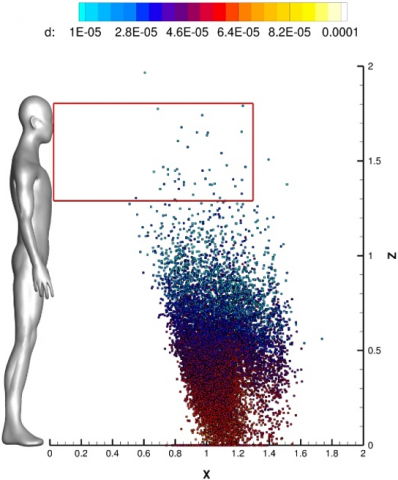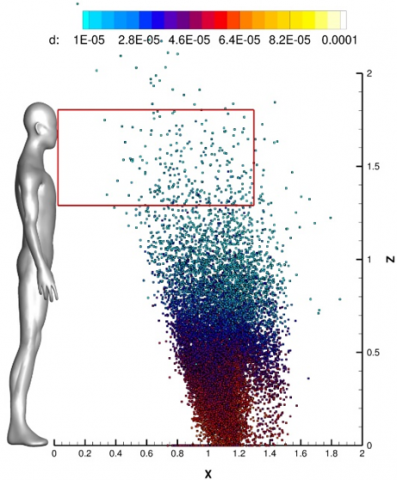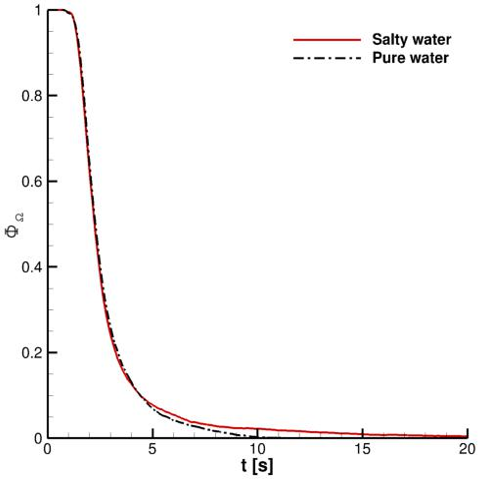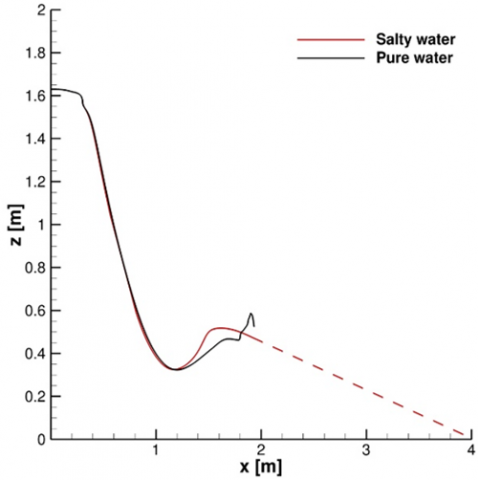# Impact of Sodium Chloride Crystallization on Saliva Droplets Spreading

Impact of Sodium Chloride Crystallization on Saliva Droplets Spreading

Valerio D’AlessandroMatteo Falone Luca Giammichele Renato Ricci

Dipartimento di Ingegneria Industriale e Scienze Matematiche, Università Politecnica delle Marche Via Brecce Bianche, 60131 Ancona (AN), Italy

Corresponding Author Email:
v.dalessandro@univpm.it
Page:
307-311
|
DOI:
https://doi.org/10.18280/ti-ijes.652-425
26 March 2021
|
Accepted:
10 May 2021
|
Published:
30 July 2021
| Citation

OPEN ACCESS

Abstract:

It is well known that SARS–CoV–2, can be transmitted through airborne diffusion of saliva droplets which travels into atmospheric air through a thermo-fluid dynamic interaction with it.

In order to limit SARS-CoV-2 spread, social distancing is crucial. However, in this context is really important to emphasize that available knowledge is largely inadequate to make predictions on the airborne spreading of infectious droplets emitted during a cough and/or sneezing.

The main aim of our research activity is to provide a contribution to thermo-fluid dyamic modeling of saliva droplets diffusion produced by coughing. In particular in this paper, several efforts were devoted to the analysis of impact of saliva chemical composition on the their spreading in space.

Keywords:

saliva droplets, crystallization kinetics, population balance equation, Eulerian-Lagrangian modeling

1. Introduction

It well known that several viruses can be transmitted through airborne diffusion of the fluids and micro-particles produced by human respiratory tracts. Typical infection mechanisms are the discussed in Mittal et al.  and they can be classified as follows: (i) direct transfer of large droplets to the receiver’s conjunctiva, mouth, or nose; (ii) physical contact with droplets deposited on the surface and subsequent absorption to the nasal mucosa of the receiver; and (iii) inhalation of respiratory ejected aerosolized droplet nuclei.

It is very easy to understand that a similar topic is of crucial interest after the unprecedented COVID-19 pandemic. Indeed, during this period many countries in the world have imposed variable social distances to be maintained between persons. However, appropriate social distancing rules have to be defined on the basis of saliva droplets physics which is not completely understood. For this reason several research groups have started research efforts in order to gain new insight into the transport of fluids and biological sprays deriving from respiratory activities.

After their emission, saliva droplets travel as a result of their inertia, their weight and their aerodynamic interaction with atmospheric air. Furthermore, the mass of each droplet can vary due to evaporation. This process, in turn, can produce a supersaturated solution of sodium chloride (NaCl) in water which are the main components of human saliva. This particular condition triggers crystallization nuclei and their growth resulting in the formation of solid dry nuclei (also known as droplet nuclei). This aspect is of great interest on the contamination risk assessment since dry nuclei have small diameters which imply long fly time.

The long term goal of this research activity is the development of a reliable computational model, relying on OpenFOAM library , for the saliva droplets’ dynamics. In particular this paper focuses on our recent efforts about modeling of NaCl crystallization kinetics, within a saliva droplets, induced by NaCl/water solution supersaturation. To this end, we present a multi-scale mathematical model that can predict droplet nuclei generation and their space evolution.

This paper is organized as follows: the governing equations are reported in Sec. 2, while the numerical approximations are discussed in Sec. 3. Numerical results are shown in Sec. 4. Finally, Sec. 5 contains the conclusions.

2. Governing Equations

The here presented numerical computations are developed using an Eulerian–Lagrangian framework briefly described in the following. In particular, the particle-source-in-cell (PSI-Cell) method  is adopted to couple Eulerian and Lagrangian phases, while Population Balance Equation (PBE) is solved at droplet level to take into account NaCl crystallization kinetics.

Eulerian phase was modeled using standard compressible Reynolds Averaged Navier-Stokes (RANS) equations. Turbulence modeling is performed using standard SST k-ω model developed by Menter  not described here for the sake of compactness.

As regards saliva particles a Lagrangian frame is adopted. It is worth noting that, within OpenFOAM Lagrangian libraries, for trivial efficiency reasons, the concept of computational parcel is adopted. This means that particles are organized in groups, called parcels, and each parcel represents the center of mass of a small cloud of particles having the same properties. Non-collisional spherical parcels are employed in our approach. Thus, position and velocity are the results of the trajectory and momentum equations. The forces acting on the particles considered in this research work are the following: gravity, aerodynamic drag and buoyancy. Aerodynamic drag coefficient is obtained from Putnam correlation . Mass and energy equations were also solved for each parcels; the convective heat transfer coefficient and the mass transfer one are obtained from the Ranz-Marshall correlation for Nusselt and Sherwood numbers .

The Rosin–Rammler distribution is used for representing initial parcels’ diameter. The curve parameters calibration are the same discussed in D’Alessandro et al. .

In order to simulate the nucleation and growth of NaCl crystals within a saliva droplet a coupling strategy between PSI-Cell method and population balance equation (PBE) is presented. In our approach PSI-Cell method is used in order to predict the particles interaction with Eulerian phase, while PBE is solved at parcel level to consider droplet nuclei generation.

PBE for spatially in-homogeneous crystallization processes can be written as follows, :

\begin{align} & \frac{\partial {{N}_{j}}}{\partial t}+\nabla \cdot \left( {{N}_{j}}{{\mathbf{u}}_{p}} \right)-\nabla \cdot \left( {{D}_{t}}\nabla {{N}_{j}} \right) \\ & =-\sum\limits_{j}{\frac{\partial \left[ {{G}_{j}}{{N}_{j}} \right]}{\partial {{r}_{j}}}}+B\prod\limits_{j}{\delta }\left( {{r}_{j}}-{{r}_{j0}} \right)+h. \\\end{align}      (1)

where, Nj is the NaCl crystals number density within a parcel, Dt is the local turbulent diffusivity, Gj is the growth rate, rj is the particle internal coordinate, rj0 is the particle internal coordinate for a crystal nucleus, δ is the Dirac function, B is the nucleation rate, and h is the creation or destruction of particles due to aggregation, agglomeration, and breakage.

It is also important to note that the PBE of a parcel includes the time derivative and particle dimensions and can ignore the spatial dimension. Moreover, in this study we refer only to crystallization phenomenon and this is the reason why PBE needs to take into account only nucleation and growth of particles.

Eq. (1) can be re-written integrating over r:

$\frac{d{{f}_{j}}}{dt}=H$       (2)

where, fj is the parcel-averaged population and H is defined as follows:

\begin{align} & H=-\frac{1}{\Delta r}{{G}_{j+1/2}}\left( {{f}_{j}}+\frac{\Delta r}{2}{{\left( {{f}_{r}} \right)}_{j}} \right) \\ & +\frac{1}{\Delta r}{{G}_{j-1/2}}\left( {{f}_{j-1}}+\frac{\Delta r}{2}{{\left( {{f}_{r}} \right)}_{j-1}} \right). \\\end{align}        (3)

Note that, the nucleation term is included in the cell, corresponding to the nuclei size, by averaging the nucleation rate.

Starting from Eq. (2) solution the parcel-averaged crystal mass can be evaluated as in Woo et al. :

${{N}_{w,j}}=\frac{1}{4}{{\rho }_{c}}{{k}_{v}}{{f}_{j}}\left( r_{j+1/2}^{4}-r_{j-1/2}^{4} \right).$      (4)

Therefore, semi-discrete PBE can be formulated as follows:

\begin{align} & \frac{d{{N}_{w,j}}}{dt}=\frac{{{\rho }_{c}}{{k}_{v}}}{4}\left( r_{j+1/2}^{4}-r_{j-1/2}^{4} \right) \\ & \times \max (\operatorname{sign}\Delta c,0)H \\\end{align}       (5)

From Eq. (5), we can also calculate the total mass related to crystallization process following Woo et al. .In the above equation Δc=c-c* is supersaturation, while c and c* are the NaCl concentration and its solubility in pure water. Nucleation and growth rates coefficients present in Eq. (5) are obtained from literature experimental data for NaCl/water solutions [9, 10].

3. Numerical Approximation

The governing equations solution relies on the unstructured, collocated, cell-centered finite volume approach available within OpenFOAM library. An implicit, three level, second-order scheme was used for the time integration together with the dynamic adjustable time stepping technique for guaranteeing a local Courant (Co) number less than a user-defined value.

Face interpolation of convective fluxes is handled by the linear upwind scheme, whereas diffusive terms are discretized by a standard second-order central scheme. Furthermore, pressure–velocity coupling is handled through the Pressure-Implicit with Splitting Operators (PISO) procedure. As regards linear solvers, a preconditioned conjugate gradient (PCG) method with a diagonal incomplete-Cholesky preconditioner was used to solve the pressure equation. A preconditioned bi-conjugate gradient (PBiCG) method with the Diagonal Incomplete Lower Upper (DILU) preconditioner was adopted instead for the remaining equations. In particular, a local accuracy of 10-7 was established for the pressure, whereas other linear systems were considered as converged when the residuals reached the machine precision.

A 3D computational domain was considered. It consists of an air volume starting from the mouth print of a standing coughing person. A length L=4 m, a width W=1 m, and a height H=3 m were adopted, in accordance with Dbouk and Drikakis . A suite of three fully structured grids, having hexahedral cells were built in order to discretize the domain. All the related details as well as the space-time convergence study can be found in our former paper .

Lagrangian phase momentum and mass equations were solved using a standard Euler scheme for time-integration. Energy equation was solved analytically. A special care was devoted to PBE which was time-integrated using an explicit Strong Stability Preserving Runge-Kutta (SSPRK) having 9 stages and 5-th order of accuracy , to avoid blow-up of the computations. Moreover, (fr)j terms, are approximated by the min-mod limiter .

3.1 Initial and boundary conditions

A stepped velocity inlet at the mouth boundary was applied to mimic the human cough over 0.12 s. The velocity inlet value was deduced on the basis of literature data and it is equal to 8.5 m/s in the stream-wise direction both for the carrier fluid and injected parcels [7, 11].

Saliva droplets were also injected into the domain from the mouth print boundary. Specifically, the initial total mass of saliva droplets laden into the domain for a single cough event is 7.7 mg, according to Dbouk and Drikakis . All the other relevant details can be found in D’Alessandro et al. .

The initial temperature of the carrier fluid is 20℃ with relative humidity fixed at 50%. The ground is at 25℃, while the air and droplets ejected by human mouth are at 34℃. No background flow is included in the presented computations. However, initial fields consistent with atmospheric conditions were adopted. These fields are obtained through a preliminary computation which does not provide Lagrangian particles into the domain. It is worth noting that cloud evolution is strongly influenced by this condition .

A key role is played by saliva chemical composition which in general a complex fluid. However, in many CFD oriented papers it is modeled as pure water. In this work we take into account the presence of NaCl within saliva droplets. In particular, we consider NaCl completely diluted in water and related concentration value are obtained from Rosti et al. .

4. Results

In this section we present results deriving from numerical computations referred to the saliva droplets produced during coughing.

Some cloud characteristics are computed in order to investigate its diffusion, i.e., (i) the cloud center of mass; (ii) fraction of particles present in a reference volume.

The cloud center of mass is defined in the following equation:

$^{-}\mathbf{G}=\frac{\sum\limits_{i=1}^{{{N}_{p}}\left( {{\Omega }_{0}} \right)}{{{m}_{P,i}}}{{\mathbf{x}}_{P,i}}}{\sum\limits_{i=1}^{p\left( {{\Omega }_{0}} \right)}{{{m}_{P,i}}}},$     (6)

where, Np0) is the number of parcels laden in the overall domain, Ω0, in a given time-instant. In the following, G=(xG , yG , zG ) is considered as the center of mass components. It is worth noting that x-axis is associated to stream-wise direction, y-axis represent the transverse direction, while z is the vertical one. On the other hand, the ratio between the number of particles presents in a reference volume, Ωi, and the total number of particles in Ω0 (in a given time instant) is used to track the droplets’ population distribution in risk zone. The reference index is defined as:

${{\Phi }_{{{\Omega }_{i}}}}=\frac{\sum\limits_{k=1}^{{{N}_{p}}\left( {{\Omega }_{i}} \right)}{{{N}_{p,k}}}}{\sum\limits_{k=1}^{{{N}_{p}}\left( {{\Omega }_{0}} \right)}{{{N}_{p,k}}}}.$      (7)

The aforementioned reference volume, Ωi, is parallelepipedal type having the following features:

${{\Omega }_{i}}=\left[ 0,{{\alpha }_{i}} \right]\times [-0.5,0.5]\times [1.3,1.8]$    (8)

The parameter αi, appearing in Eq. (8), spans the following values: 1.0m and 1.3 m. Ωi stream-wise dimension was selected in order to investigate the effectiveness of 1 m distance, which is the safety distance adopted in Italy. The transverse direction range is considered in order to completely cover the domain. The z-axis interval is defined for acting on a sufficiently wide range of possible virus receivers’ heights.

In this paper we address saliva chemical composition effect. More in depth, we discuss the impact of NaCl presence within droplets, rather than pure water particles, on the related cloud space-time evolution. In this framework, looking at Figure 1 and Figure 2, it is very easy to note that at t=10 s the effect of NaCl crystallization process produces an evident impact on the parcels’ space distribution. In particular, the risk area is sensibly more populated, even for a stream-wise length grater than 1 m, when salty droplets are considered. This evidence is also expressed quantitatively from ΦΩ time-history represented in Figure 3. It is also very important to put in evidence that the particles contained into Ωi domain are dry and they have diameters approximately around 10 μm. For this reason a fly time having an order of magnitude of 60 s is expected.

## 1.pngFigure 1. Cloud representation at t=10s. Parcels are colored with the particle diameter. Pure water dropltes

## 2.pngFigure 2. Cloud representation at t=10s. Parcels are colored with the particle diameter. Salty water dropltes

The center of mass trajectory on the x–z plane is shown in Figure 4. For 0.35m<xG<1m, the xG, zG curve underlines a peculiar trend. Actually, the trajectory is almost linear due to the cancellation of the inertial term. For xG>1 m the impact of droplets chemical composition is evident and it is related to the crystallization effects and the consequent droplet nuclei generation. For xG>2 m case the curve zG=f(xG) is extrapolated since the background flow is extinct and the Lagrangian particles have uniform velocity. It is really evident that dry nuclei are able to reach distance sensibly longer than pure water droplets which completely evaporates from the domain in less than 20 s.

5. Conclusions

This paper addresses a preliminarily assessment of saliva droplets chemical composition on their spreading in moist air.

## 3.pngFigure 3. Fraction of particles present in the volume Ωi

## 4.pngFigure 4. Saliva cloud center of mass evolution

For this reason, we have also introduced a multi-scale mathematical model to predict NaCl crystallization kinetics. In particular, a coupling approach between PSI-Cell method and PBE was proposed. In this context, it was showed that the chemical composition of saliva droplets laden into CFD domain strongly affects the obtained solution. Specifically, the NaCl crystallization produces dry particles having small diameters which can be transported for time longer than pure water droplets.

Future work will be devoted to more realistic configurations in several relative humidity conditions since this parameter strongly affect evaporation phenomena hence crystallization kinetics.

Acknowledgements

The authors want to acknowledge “Associazione Nazionale Big Data” that awarded this research work within COVID-19–Fast access to the HPC supercomputing facilities program. We acknowledge ENEA for awarding us access to CRESCO6 based at Portici.

References

 Mittal, R., Ni, R., Seo, J.H. (2020). The flow physics of COVID-19. Journal of fluid Mechanics, 894. https://doi.org/10.1017/jfm.2020.330

 Weller, H.G., Tabor, G., Jasak, H., Fureby, C. (1998). A tensorial approach to computational continuum mechanics using object-oriented techniques. Computers in Physics, 12(6): 620-631. https://doi.org/10.1063/1.168744

 Crowe, C.T., Sharma, M.P., Stock, D.E. (1977). The particle-source-in cell (PSI-CELL) model for gas-droplet flows. Journal of Fluids Engineering, 99: 325-332. https://doi.org/10.1115/1.3448756

 Menter, F.R. (1994). Two-equation eddy-viscosity turbulence models for engineering applications. AIAA Journal, 32(8): 1598-1605. https://doi.org/10.2514/3.12149

 Putnam, A. (1961). Integratable form of droplet drag coefficient. Ars Journal, 31(10): 1467-1468.

 Ranz, W.E., Marshall, W.R. (1952). Evaporation from drops. Chemical Engineering Progress, 48: 141–146.

 D'Alessandro, V., Falone, M., Giammichele, L., Ricci, R. (2021). Eulerian–Lagrangian modeling of cough droplets irradiated by ultraviolet–C light in relation to SARS-CoV-2 transmission. Physics of Fluids, 33(3): 031905. https://doi.org/10.1063/5.0039224

 Woo, X.Y., Tan, R.B., Chow, P.S., Braatz, R.D. (2006). Simulation of mixing effects in antisolvent crystallization using a coupled CFD-PDF-PBE approach. Crystal Growth & Design, 6(6): 1291-1303. https://doi.org/10.1021/cg0503090

 Desarnaud, J., Derluyn, H., Carmeliet, J., Bonn, D., Shahidzadeh, N. (2014). Metastability limit for the nucleation of NaCl crystals in confinement. The Journal of Physical Chemistry Letters, 5(5): 890-895. https://doi.org/10.1021/jz500090x

 Naillon, A., Joseph, P., Prat, M. (2017). Sodium chloride precipitation reaction coefficient from crystallization experiment in a microfluidic device. Journal of Crystal Growth, 463: 201-210. https://doi.org/10.1016/j.jcrysgro.2017.01.058

 Dbouk, T., Drikakis, D. (2020). On coughing and airborne droplet transmission to humans. Physics of Fluids, 32(5): 053310. https://doi.org/10.1063/5.0011960

 Ruuth, S.J., Spiteri, R.J. (2004). High-order strong-stability-preserving Runge--Kutta methods with downwind-biased spatial discretizations. SIAM Journal on Numerical Analysis, 42(3): 974-996. https://doi.org/10.1137/S0036142902419284

 Rosti, M.E., Olivieri, S., Cavaiola, M., Seminara, A., Mazzino, A. (2020). Fluid dynamics of COVID-19 airborne infection suggests urgent data for a scientific design of social distancing. Scientific Reports, 10(1): 1-9. https://doi.org/10.1038/s41598-020-80078-7